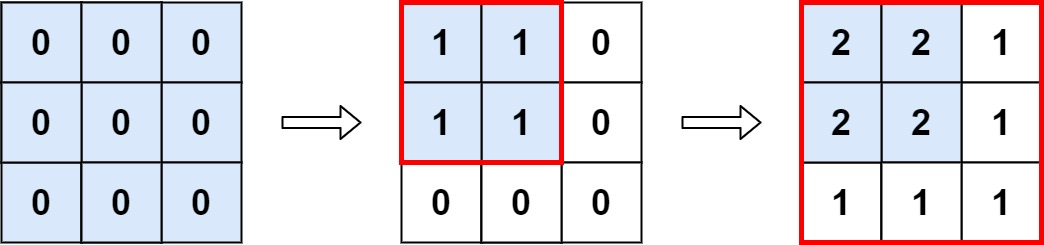# Leetcode 598 - Range addition II

Note:

• Find the overlap of all rectangles.

Question:

You are given an m x n matrix M initialized with all 0’s and an array of operations ops, where ops[i] = [ai, bi] means M[x][y] should be incremented by one for all 0 <= x < ai and 0 <= y < bi.

Count and return the number of maximum integers in the matrix after performing all the operations.

Example:Code: